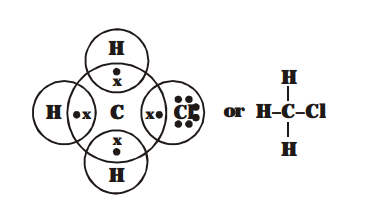# Explain the nature of the covalent

Question.
Explain the nature of the covalent bond using the bond formation in $\mathrm{CH}_{3} \mathrm{Cl}$.

solution:
Atomic number of C, H & Cl are 6, 1 & 17 respectively.

Their electronic configurations are

Carbon – 2, 4

Hydrogen – 1

Chlorine– 2, 8, 7

As C need 4 electrons to complete its octet, H needs 1 electron to complete its duplet and Cl needs 1 electron to complete its octet.

So, $\mathrm{C}$ share its 4 electron, one electron each with $3 \mathrm{H}$ atom \& 1 electron with $\mathrm{Cl}$ atom. Thus chloromethane contain $3 \mathrm{C}-\mathrm{H}$ and one $\mathrm{C}-\mathrm{Cl}$ covalent bond